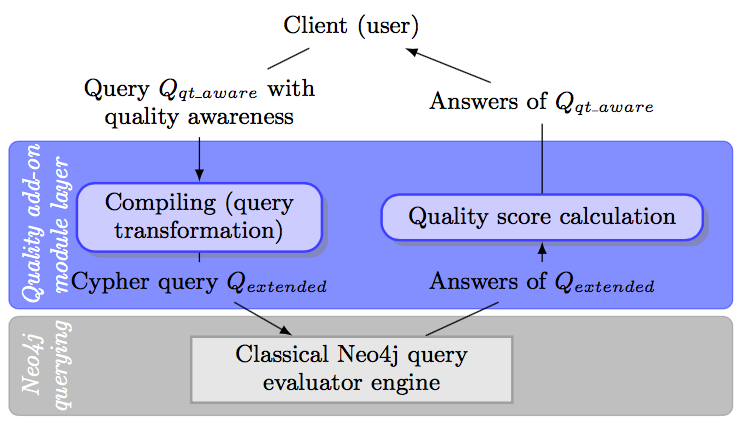# The Tamari (Quality Alerts Management in Graph Databases using Rabbithole) prototype

Tamari is a research prototype based on a theoritical general framework (under submission), which allows introducing quality awareness in graph database querying.

The graph pattern proposed in the paper can be expressed in Cypher by the following query

``````QTAWARE
MATCH (c)-[l:likes]->(w1:Work),(w1)-[:author]->(a1),(a2)-[:inspired_by]->(a1),(w2)-[:author]->(a2)
WHERE c.name='Clovis'
RETURN c,w1,a1,a2,w2``````

This is the default query embedded in the Tamari tool. The Keyword `QTAWARE` specifies that the query is quality aware (just delete the keyword in order to disable the feature).

## The Tamari Architecture

Tamari adds a quality add-on layer on  top of the classical Cypher query engine, by extending the RabbitHole console. The architecture of Tamari is depicted below.The Compiling module translates the Cypher graph pattern query into an extended one that retrieves all the needed quality information, from the profile, the quality vocabulary and the association of the vocabulary to the answers. The extented query is then sent to the (classical) Neo4j engine. Based on its answers, the Quality score calculator module calculates the quality alert scores associated to each answer.

• Tamari does not embed the full original Ne4j console Rabbithole as it is a proof-of-concept of a research work in progress, which focuses on extending the match operator.
• The software is still a prototype. For the moment, only one quality profile is considered and the considered level of interest in the quality vocabulary is the dimension. All the quality dimensions appearing in the answer are retrieved; if a dimension is not retrieved then it means that no quality alert is associated with it (ie. its alert score is 0 for all the answers).
• If you have any suggestion for improving this prototype or if you find a bug in the prototype, please do not hesitate to contact us.

In order to install and run locally the prototype on your computer, you have to

2. Enter in the tamari_0.9 folder (`cd tamari_0.9`)
3. Build the project with Maven (which should be previously installed on your computer) by `mvn clean install exec:java`
4. Open the application in a web browser at http://localhost:8080

## The Tamari Interface

The figure below is a screenshot of the application. The interface is composed of two frames:

1. a central frame for visualizing the graph and results of a query, the data graph is overprinted on, you can hide it by clicking on the Toggle Viz button;
2. a small frame under the central one, for writing a query (click on the Run button to execute the query).

When a query is executed, a table containing the results appears in the central frame (a row in this table is a subgraph answer) and nodes of the graph that belong to the answer are colored in red.Logs trace the evaluation in an associated console, providing each intermediate result of the execution process viz. the extended query obtained after the compiling stage (output of the Compiling module), its results (intermediate result containing additional information needed for the quality alert scores calculation), and the final result obtained after the calculation of the quality alert scores (output of the Score Calculator module), which is also user-friendly printed on the central frame of the GUI.

For instance, one can see in such a log trace that the extended query associated with the default query is

``````MATCH (c)-[l:likes]->(w1:Work),(w1)-[:author]->(a1),(a2)-[:inspired_by]->(a1),(w2)-[:author]->(a2)
OPTIONAL MATCH (a1)<-[:Subgraph]-(:Subgraph)-[_qt_a1_ass:association]-(_qt_a1_tag:Voc), _qt_pa1=(_qt_a1_tag)<-[*]-(_qt_a1_level_interest:Voc{type:'dimension'})
OPTIONAL MATCH (a2)<-[:Subgraph]-(:Subgraph)-[_qt_a2_ass:association]-(_qt_a2_tag:Voc), _qt_pa2=(_qt_a2_tag)<-[*]-(_qt_a2_level_interest:Voc{type:'dimension'})
OPTIONAL MATCH (c)<-[:Subgraph]-(:Subgraph)-[_qt_c_ass:association]-(_qt_c_tag:Voc), _qt_pc=(_qt_c_tag)<-[*]-(_qt_c_level_interest:Voc{type:'dimension'})
OPTIONAL MATCH (w1)<-[:Subgraph]-(:Subgraph)-[_qt_w1_ass:association]-(_qt_w1_tag:Voc), _qt_pw1=(_qt_w1_tag)<-[*]-(_qt_w1_level_interest:Voc{type:'dimension'})
OPTIONAL MATCH (w2)<-[:Subgraph]-(:Subgraph)-[_qt_w2_ass:association]-(_qt_w2_tag:Voc), _qt_pw2=(_qt_w2_tag)<-[*]-(_qt_w2_level_interest:Voc{type:'dimension'})
WITH  _qt_a2_level_interest AS _qt_a2_level_interest,  w1 AS w1,  _qt_a1_ass,  _qt_a2_ass,  a1 AS a1,  _qt_a1_tag AS _qt_a1_tag,
REDUCE(weight = 1, x IN relationships(_qt_pa2)| weight * x.w) AS _qt_a2_weight,
REDUCE(weight = 1, x IN relationships(_qt_pa1)| weight * x.w) AS _qt_a1_weight,
_qt_w2_level_interest AS _qt_w2_level_interest,  _qt_c_tag AS _qt_c_tag,  _qt_c_ass,
_qt_a1_level_interest AS _qt_a1_level_interest,  _qt_w2_ass,  _qt_w1_level_interest AS _qt_w1_level_interest,
w2 AS w2, REDUCE(weight = 1, x IN relationships(_qt_pw2)| weight * x.w) AS _qt_w2_weight,
c AS c,  _qt_c_level_interest AS _qt_c_level_interest,  _qt_w1_ass,  _qt_a2_tag AS _qt_a2_tag,
REDUCE(weight = 1, x IN relationships(_qt_pc)| weight * x.w) AS _qt_c_weight,  _qt_w2_tag AS _qt_w2_tag,  _qt_w1_tag AS _qt_w1_tag,
REDUCE(weight = 1, x IN relationships(_qt_pw1)| weight * x.w) AS _qt_w1_weight,  a2 AS a2 WHERE  c.name='Clovis'
RETURN DISTINCT _qt_a1_ass.level, _qt_a1_level_interest.name, _qt_a1_tag, _qt_a1_weight, _qt_a2_ass.level, _qt_a2_level_interest.name, _qt_a2_tag, _qt_a2_weight, _qt_c_ass.level, _qt_c_level_interest.name, _qt_c_tag, _qt_c_weight, _qt_w1_ass.level, _qt_w1_level_interest.name, _qt_w1_tag, _qt_w1_weight, _qt_w2_ass.level, _qt_w2_level_interest.name, _qt_w2_tag, _qt_w2_weight, a1, a2, c, w1, w2``````